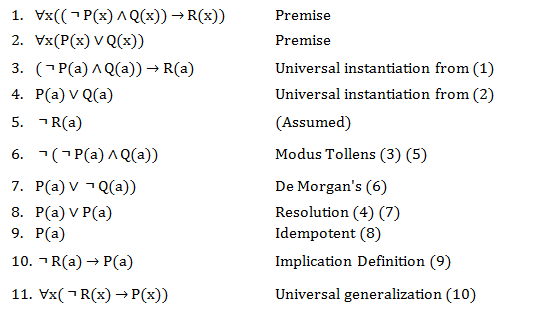# Discrete Math, Intro Proof

• ParoXsitiC

## Homework Statement

Using the rules of inference, prove that if ∀x(P(x) ∨ Q(x)) and ∀x((￢P(x) ∧ Q(x)) → R(x)) are true, then ∀x(￢R(x) → P(x)) is true as well.

## The Attempt at a SolutionThe problem arises step 5. I feel this is correct but the instructor has stated that assuming in a proof:

"you are not allowed to assume any more information that what is given. Otherwise, you are solving a different problem."

I felt since my assumption is a bit like a scenario in a truth table that it was OK, because it does not contradict any of the premises.

I have no idea how to approach this problem other than this.

Anytime you are asked to prove an implicated statement, in this case, "(Not R) imples P", you may assume the first part to prove the second part. In this case, your professor has added additional stipulations that you must assume as well, so I'd argue that 5 is not only valid, but entirely necessary for proving what you're asked.

A->B is equivalent to it "contrapositive", -B->A. Since R is the conclusion of the statement to be proved, -R is the hypothesis of its contrapositive.

Anytime you are asked to prove an implicated statement, in this case, "(Not R) imples P", you may assume the first part to prove the second part. In this case, your professor has added additional stipulations that you must assume as well, so I'd argue that 5 is not only valid, but entirely necessary for proving what you're asked.

The problem is not to prove ∀x(￢R(x) → P(x)) but rather to show that it follows from ∀x(P(x) ∨ Q(x)) and ∀x((￢P(x) ∧ Q(x)) → R(x)). Assuming ￢R(x) to be true is not necessary and not valid in the kind of proof that is being asked for.

One person said it's OK. Another said it's not. And one just gave just a general definition. If I cannot assume -R(a) is true then I need a push in the right direction on how to solve this without using this assumption, or at least a hint on how I can proof -R(a) is true using rules.

ParoXsitiC said:
One person said it's OK. Another said it's not. And one just gave just a general definition. If I cannot assume -R(a) is true then I need a push in the right direction on how to solve this without using this assumption, or at least a hint on how I can proof -R(a) is true using rules.

You're not supposed to assume or prove that ￢R(a) is true, you're suppose to show ￢R(x) → P(x) is true for all x whenever ∀x(P(x) ∨ Q(x)) and ∀x((￢P(x) ∧ Q(x)) → R(x)) are true. I don't know what rules of inference you are permitted to use, so I can't tell you exactly how you should proceed, but I think HallsofIvy's hint, that you should try proving the contrapositive, might be the way to go.

Edit: If you have an axiom that says something along the lines of ((A→B)∧￢B)→￢A, you may be able to salvage your proof.

Last edited:
I would argue that taking the first two conditions along with not R is perfectly valid in this problem, as the question is ultimately "given these two conditions, prove this implication" and how you prove an implication is to assume the premise. However I do admit it's less formal and more a tool for someone who really knows what they're doing. Since discrete structures aims to teach basic understandings of proof structures, with a great emphasis on formality and distinctive rules, what the professor is probably aiming for is using the contrapositive of  and combining it with  through .